Industrial Data Science
in C# and .NET:
Simple. Fast. Reliable.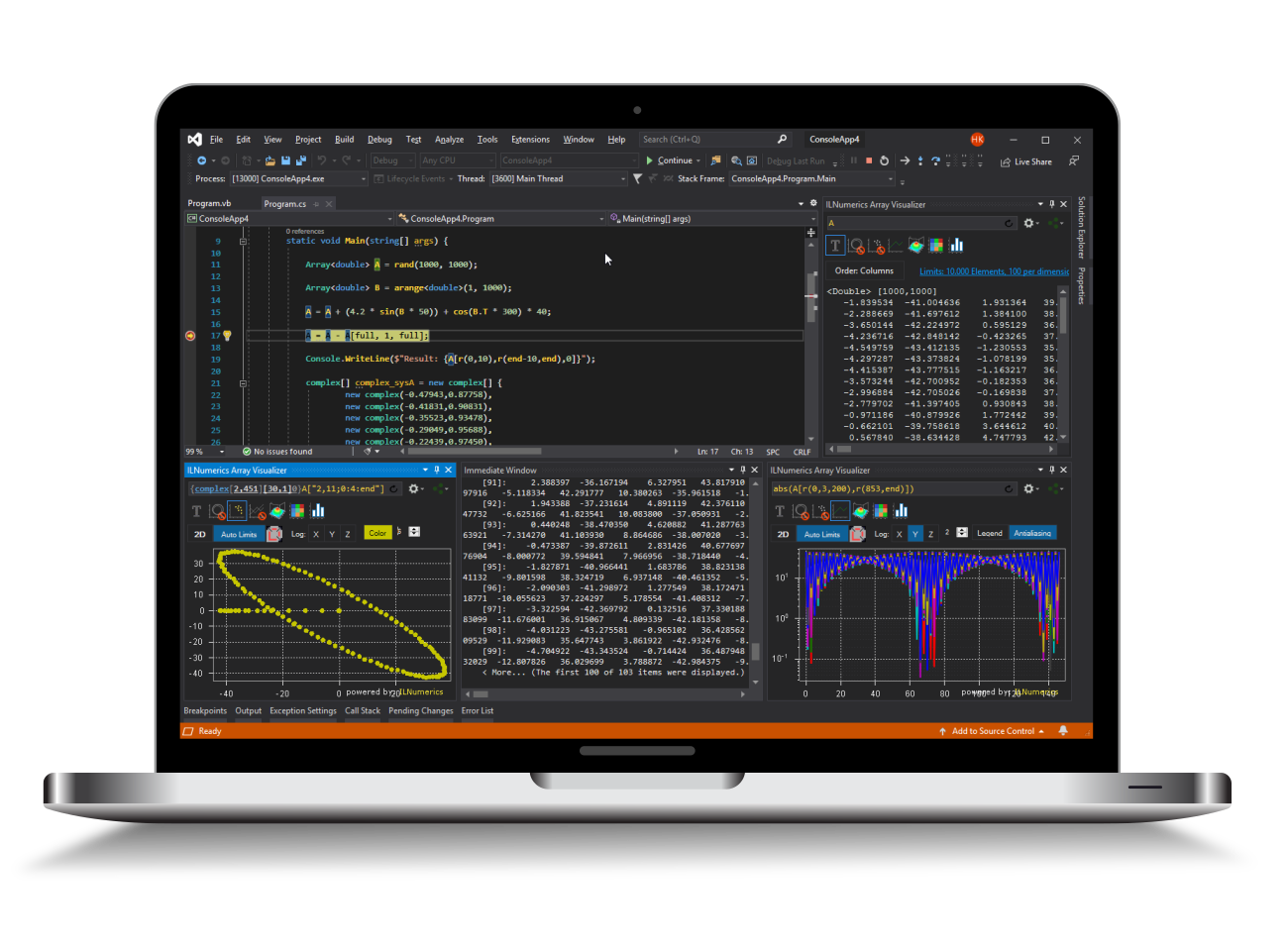ILNumerics - Technical Computing

Modern High Performance Tools for Technical

Computing and Visualization in Industry and Science

# Plotting Types for Debug Data

The ILNumerics Array Visualizer is a time saving tool for Visual Studio. During your debug session it connects to the Visual Studio Debug Engine and allows to visualize the results of arbitrary expressions: variables, arrays, pointers. Results are plotted in various ways as 2D and 3D charts, following updates to your data in each debug step automatically. The Array Visualizer is available for C#, Visual Basic, F#, C/C++ and FORTRAN, for 32/64 bit. General introductory documentation is found here. This article introduces the various plotting options for visualizing your debug data.

## Plotting Types

The Array Visualizer enables individual plot types depending on the shape of the expression result. Enabled types can be selected from the top row of the Array Visualizer tool window. Types which are not available for the current array expression result are grayed out and marked with a red slashed circle sign.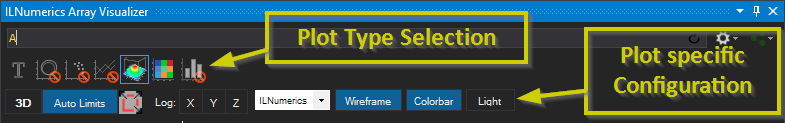The following types are available:

### Text Output

The text ouput is the default output type. It gives a simple string representation of the data and is always available - independently from the current shape of your debug data. Multidimensional arrays are displayed as 2D slices along the first or last 2 dimensions, in a tabular format. Text output serves as a fallback for all expressions, which cannot be visualized by any graphical means.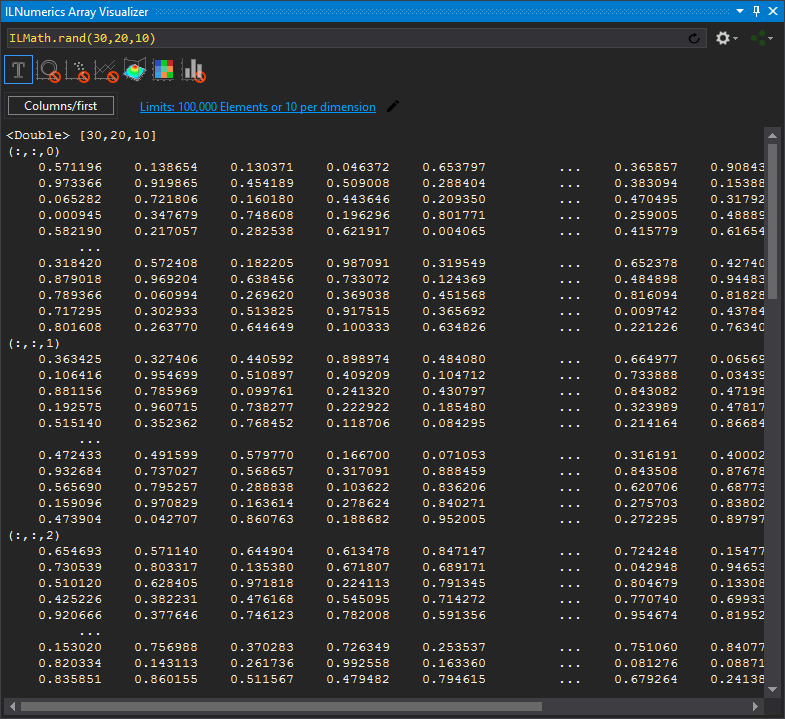### Line Output

Available for row and column vectors, matrices of 1...3 rows. A single line is displayed as Y, X-Y or X-Y-Z plot - depending on the number of rows provided.

Example:

Let A be an ILNumerics array of size (3 x 20) elements. The expression 'A(0,full)' corresponds to a vector with 20 elements. It enables the line plot type and plots the first row of A: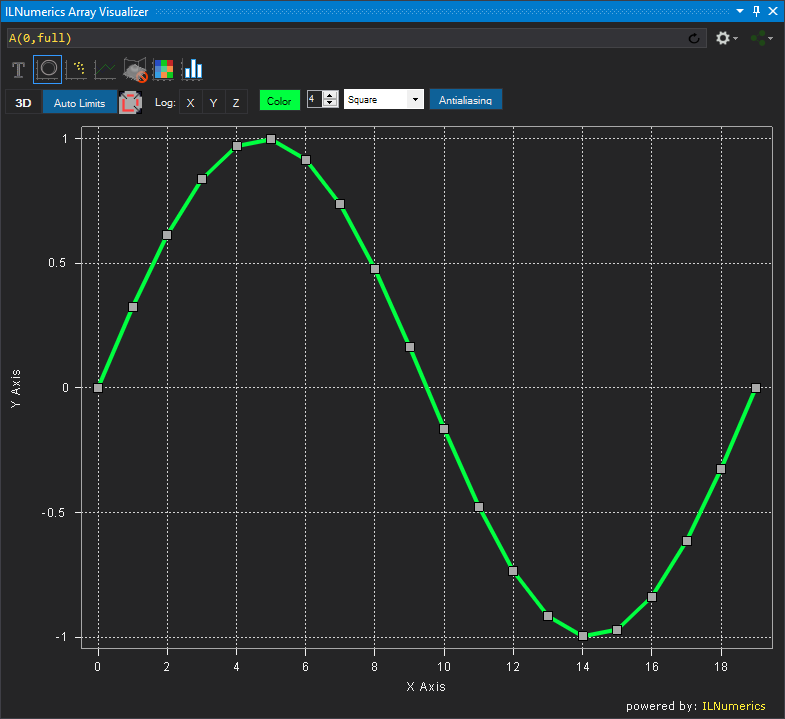Line plots are commonly used to plot lines for data of up to 3 coordinates. ILNumerics arrays subarray feature allows to pick arbitrary combinations of rows for combining them in XY / XZ / YZ plots. For example, we could plot the X and the Z coordinates by selecting the first and the 3rd row: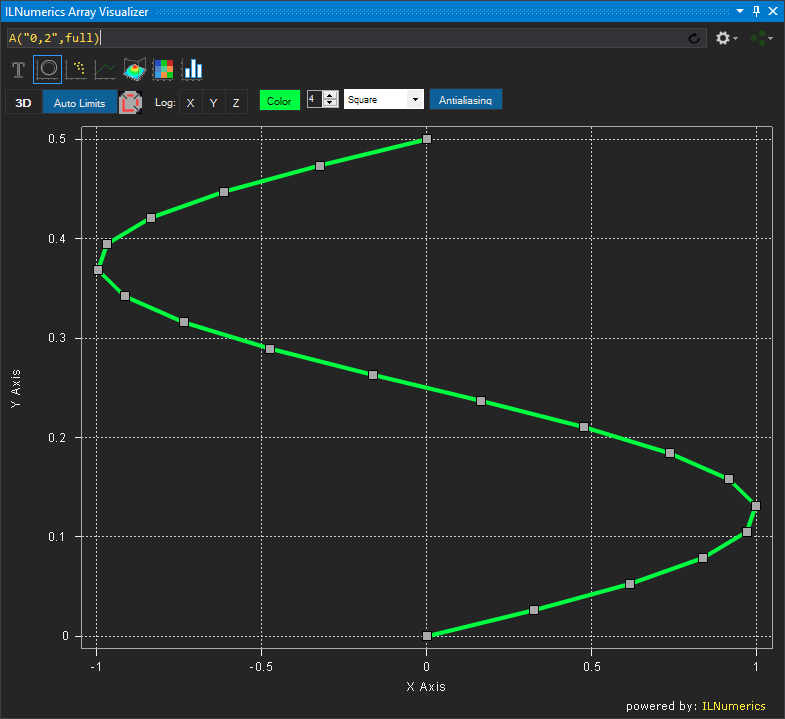XYZ plots are created when 3 rows are provided: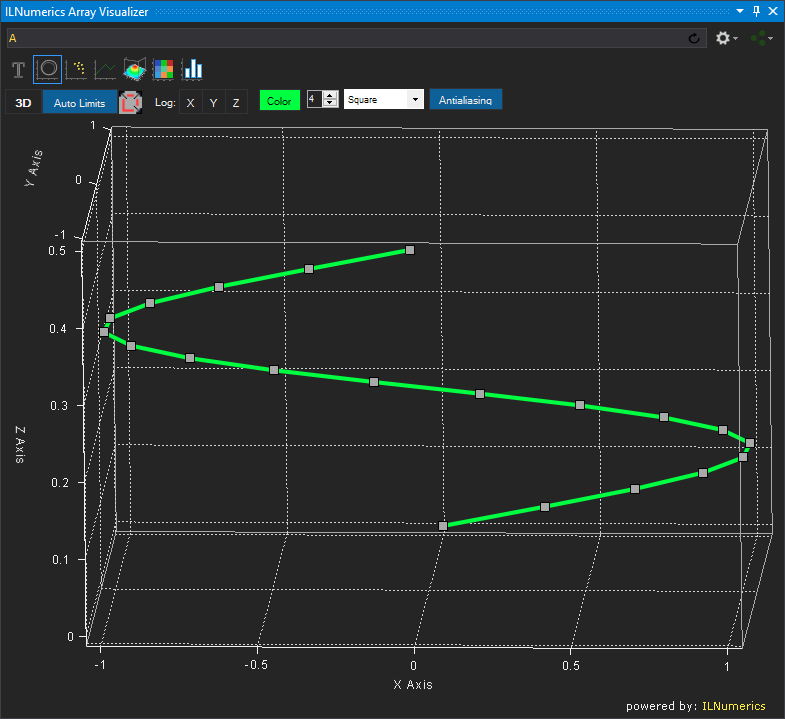ILNumerics subarrays makes is very easy to pick the needed data from your variables and to bring them into a shape, suitable for plotting with Line Plots. Other array variables, like System.Array, pointers, etc. can achieve the same results by utilizing the Array Descriptor.

### Scatter Plot

Available for matrices of size 1xn (single row), 2xn and 3xn. Individual points are drawn at the 3D positions according to the array data found in the columns of the provided matrix. For single row vectors, the data represent the X coordinates, Y and Z data are optional and taken from the 2nd and 3rd row: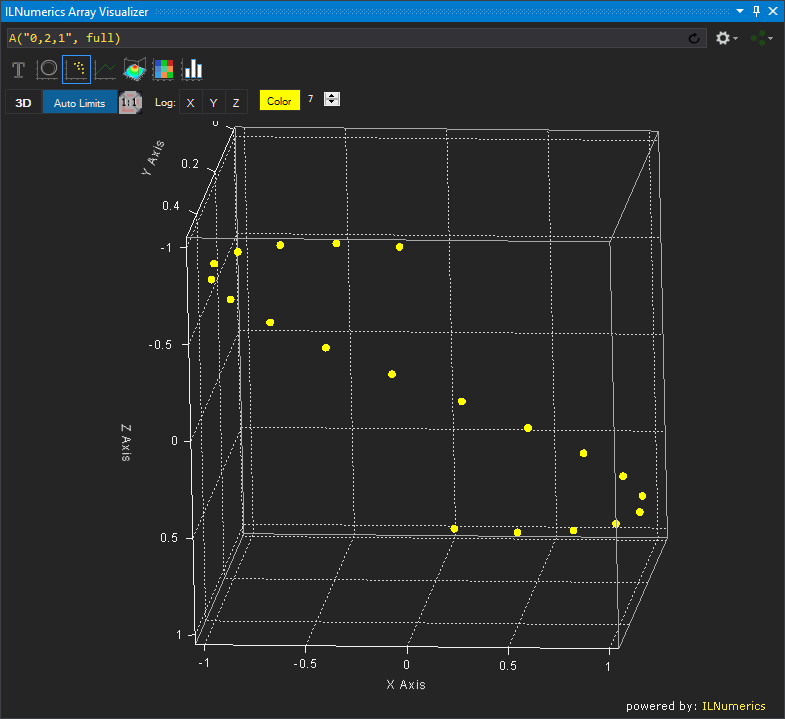ILNumerics subarrays makes is very easy to pick the needed data from your variables and to bring them into a shape, suitable for plotting with Line Plots. In the above example we have used the subarray string "0,2,1" in order to map the X,Y,Z coordinates to the 0,2,1 rows of the result. Hence, the 2nd row becomes the Z coordinate of the plot. The 3rd row acts as the Y coordinate. Other array variables, like System.Array, pointers, etc. which do not have such subarray feature can achieve similar results by utilizing the Array Descriptor.

### Multiple Lines Plot

Available for non-empty matrices. The rows of the matrix are visualized as individual line plots. By default, the Array Visualizer limits the number of lines displayed by 50. This number is configurable in the ILNumerics section of the Visual Studio options dialog.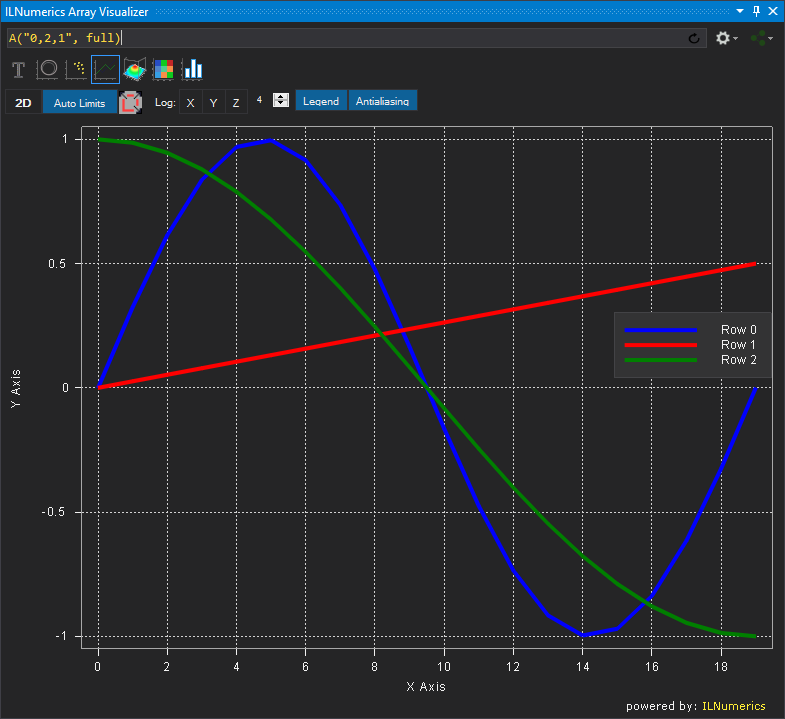Again, an ILNumerics subarray was used on the ILNumerics array variable A to swap the Y and Z coordinates stored in the second and 3rd row of A. Hence, the 2nd row becomes the Z coordinate of the plot. The 3rd row acts as the Y coordinate. Other array variables, like System.Array, pointers, etc. which do not have such subarray feature can achieve similar results by utilizing the Array Descriptor.

### Surface Plot

Available for non-empty, non-vector sized matrices, i.e. matrices with at least two rows and two columns. The matrix is displayed as surface plot. Each element of the matrix represents a grid point of the surface, intermediate tile areas between grid points are linearly interpolated.